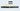# Single Number - Leet Code Solution

September 01, 2020## Problem Statement

Given a non-empty array of integers, every element appears twice except for one. Find that single one.

Example

``````Input: [2,2,1]
Output: 1

Input: [4,1,2,1,2]
Output: 4``````

## Solution using Extra Memory

We can take help of `HashMap` where we can save numbers and their counts.

• In first iteration, just fill this `HashMap`
• Iterate over this `HashMap`, and find the number whose count is not 2

### Code

``````public int singleNumber_extraMemory(int[] nums) {
int l = nums.length;

Map<Integer, Integer> map = new HashMap<Integer, Integer>();
for (int i=0; i<l; i++) {
Integer count = map.get(nums[i]);
if (count == null) {
count = 1;
}
else {
count ++;
}

map.put(nums[i], count);
}

for (Integer key : map.keySet()) {
if (map.get(key) != 2) {
return key;
}
}
return -1;
}``````

### Complexity

The complexity of above code is `O(n)`

## Solution using Sorting

If we can sort the numbers, then we will have all pair of numbers adjascent to each other.
Then, we can check pair of numbers where they are not equal.

### Code

``````public int singleNumber_sort(int[] nums) {
int l = nums.length;

Arrays.sort(nums);
int i=0;
while (i<l-1) {
if (nums[i] != nums[i+1]) {
return nums[i];
}

i += 2;
}
//check for last element
if (l % 2 == 1) {
return nums[l-1];
}
return -1;
}``````

### Complexity

Since we are sorting, and iterating over array once. The complexity is `O(nlogn)`

## Solution using Simple Maths

Assuming our array has all pairs. i.e. we don’t have a single number. Then, following is true:

``2 (sum of unique numbers) = (sum of all numbers)``

Now, if we know one of the number is missing in pairs. Following must be true:

``2 (sum of unique numbers) - (sum of all numbers) = Missing Number``

Lets take a look at example:

``````#input [2, 2, 1]

2 * (2 + 1) - (2 + 2 + 1)
= 6 - 5
= 1
Result = 1

#Another example
# input [4,1,2,1,2]
2 * (4 + 1 + 2) - (4+1+2+1+2)
= 14 - 10
= 4
Result = 4``````

### Code

``````public int singleNumber(int[] nums) {
int l = nums.length;
Set<Integer> set = new HashSet<>();

int sum = 0;
int uniqueElementSum = 0;

for (int i=0; i<l; i++) {
if (!set.contains(nums[i])) {
uniqueElementSum += nums[i];
}

//all sum
sum += nums[i];
}

return 2 * uniqueElementSum - sum;
}``````

We require a `HashSet` just to check for unique elements.

### Complexity

The complexity is `O(n)`